# E-PolyLearning

 1. Find out which of the figures (1), (2), (3) and (4) can be formed from the pieces given in figure (X).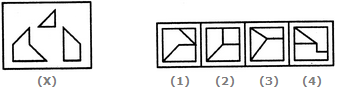a. 1 b. 2 c. 3 d. 4

 2. Find out which of the figures (1), (2), (3) and (4) can be formed from the pieces given in figure (X).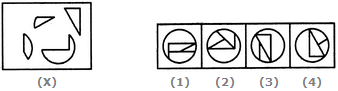a. 1 b. 2 c. 3 d. 4
 3. Find out which of the figures (1), (2), (3) and (4) can be formed from the pieces given in figure (X).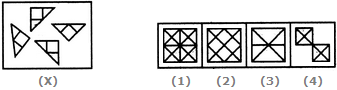a. 1 b. 2 c. 3 d. 4
 4. Find out which of the figures (1), (2), (3) and (4) can be formed from the pieces given in figure (X).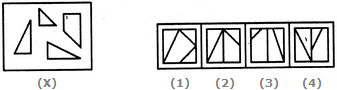a. 1 b. 2 c. 3 d. 4
 5. Find out which of the figures (1), (2), (3) and (4) can be formed from the pieces given in figure (X).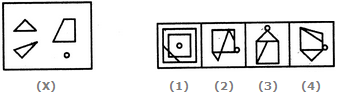a. 1 b. 2 c. 3 d. 4